Multivariate Analysis Matrix Operations

The Matrix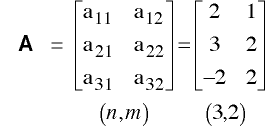• An element of A is aij
• aij is called the ijth element of A

Multiplication by a Scalar

• Multiply each element of the matrix by the scalar.

• Let c = 3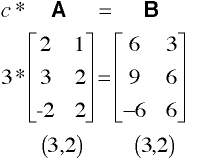Matrix Addition & Subtraction

• Add each element of the first matrix to the corresponding element of the second matrix.

• In order to add matrices, the matrices must have the same dimensions.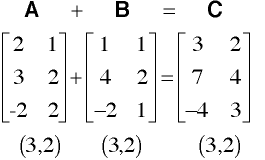• Note: A+B = B+A -- commutative law

• Note: A+(B+C) = (A+B)+C -- associative law

Matrix Multiplication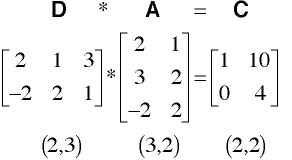Notes

• Note: In general, A*B does not equal B*A -- matrix multiplication is not commutative

• Note: (A*B)*C = A*(B*C) -- associative law

• Note: A*(B+C) = A*B + A*C
• And: (B+C)*A = B*A + C*A -- distributive law

Transpose of a Matrix

• Mathematical notation -> X'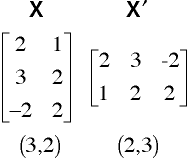Notes

• (A')' = A

• (A*B)' = B' * A'
Note the reverse order of the matrix multiplication

Column Vectors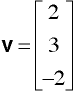Row Vector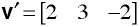Unit Vector

• Denoted as u or 1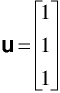Vertical MatrixHorizontal Matrix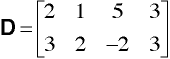Unit Matrix

• Denoted as U or 1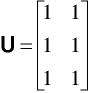Square Matrix

• Note: The number of rows equals the number of columns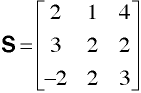Diagonal Matrix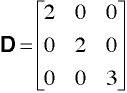Identity Matrix

• Denoted as I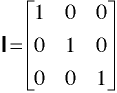• Remember I*A = A*I = A

Symmetric Matrix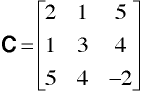• Remember C = C'

Triangular Matrix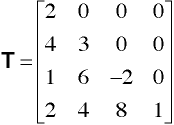Inverse of a Matrix

• Mathematical notation: B = A-1

-must be a square matrix

• Remember A-1 * A = A * A-1 = I

• In scalar terms: a-1 = 1/a

And 1/a * a = a * 1/a = 1

Inversion by Hand

Let's find the inverse of this matix: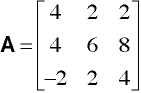First we will augment Matrix A with an identity matrix and call the result A0.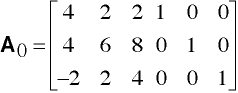We will also need an identity matrix called I0.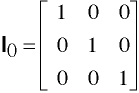Step 1

Now we will work on the first column of I0. The diagonal element in the first column of A0 is called the pivot. We will replace the first diagonal element of I0 with 1/a11. Then we will replace the non-pivot elements with -aij/a11 and call the result I1.
Compute A1 = I1 * A0.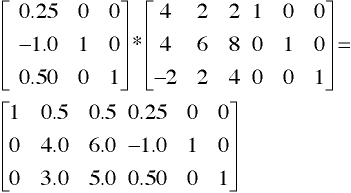Step 2

Now we repeat the process by modifying the second column of I0. This time the pivot value is the 22 element. The new matrix is called I2.
Compute A2 = I2 * A1.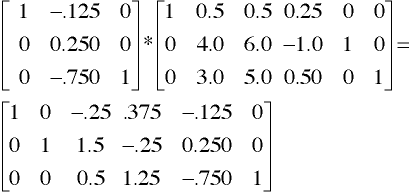Step 3

Lastly we repeat the process by modifying the third column of I0. This time the pivot value is the 33 element. The new matrix is called I3.
Compute A3 = I3 * A2.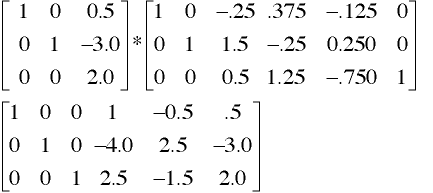The last three columns of A3 are the inverse of A, thus: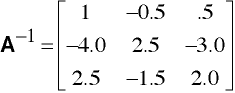Mata Example:

i=I(3)
a = (4,2,2\4,6,8\-2,2,4)
a

a = a,i
a

i1 = (.25,0,0\-1,1,0\.5,0,1)
i1

i2 = (1,-.125,0\0,.25,0\0,-.75,1)
i2

i3=(1,0,.5\0,1,-3\0,0,2)
i3

ainv = i3*i2*i1*a
ainv

ainv = ainv[1..., 4..6]
ainv

Determinant of a Matrix

• The fundamental geometric meaning of the determinant is as the scale factor for volume when matrix A is regarded as a linear transformation.

• Mathematical notation: d = |A|

Example: Inverse & Determinant of a Matrix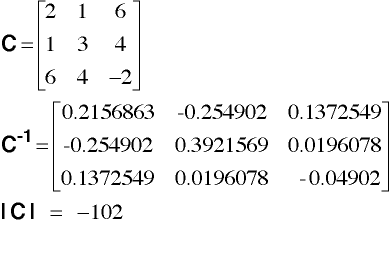Computing Column & Row Sums

• Column Sums:
• Using matrix arithmetic: T = U'*X
U'   *   X   =   T
[1 1 1] * |3 1|
|2 0| = [7 5]
|2 4|

(1,3)    (3,2)   (1,2)

• Row Sums:
• Using Matrix arithmetic: T = X*U
X   *  U   =  T
|3 1|   |1|    |4|
|2 0| * |1| =  |2|
|2 4|          |6|

(3,2)  (2,1)  (3,1)

Horizontal Concatenation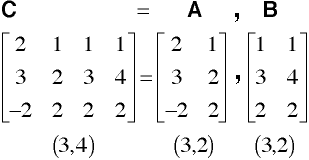Vertical Concatenation (Appending)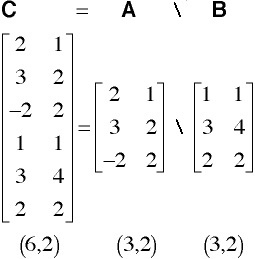Multivariate Course Page

Phil Ender, 11oct05, 30Jun98